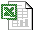Teaching Optimal Design of Experiments Using Spreadsheets

Peter Goos and Herlinde Leemans

1. Introduction

A spreadsheet turns out to be a simple and efficient tool to introduce students to the optimal design of experiments.  The calculations proceed in a number of steps, which help students to understand the problem and the solution, thereby avoiding the black-box approach often found in statistical software programs.  Our approach as well as student experiences are discussed in the paper "Teaching Optimal Design of Experiments Using a Spreadsheet", which has been published in the Journal of Statistics Education [Online], 12(3)

The following spreadsheets contain the example models described in the paper.  In order to introduce the concept of optimal design, we have concentrated on a number of well-known problems, in particular the problems of designing an experiment for estimating a simple linear regression model, quadratic regression models on one and two variables, a first order mixture model and a non-linear model.  The spreadsheets used for calculating these designs may be downloaded from this page (click the Excel icon).  For each model, we briefly explain how it can be used during the class.

We have used Excel and the standard Excel Solver to illustrate our approach.  The method is however not limited to Excel only since Solver is also available for Lotus and Quattro Pro.

a.Simple linear model (see Section 2)

The design matrix, the information matrix and the variance-covariance matrix of the parameter estimators for this problem are constructed following the steps in the paper.  We start from a given scenario (which has been saved as "start").  Students may try and find the optimal solution manually by changing the values of the design points.  We have used the Solver to find the optimal solution, which has been saved as the scenario "optimum".

b.Single-variable quadratic model (see Section 3.1)

Quadratic models are more complex than linear models, which makes it harder for students to find the optimal solution by hand.  Moreover, this problem, involving only one experimental factor, has different local optima.  Students may find "an" optimum by using the Solver, starting from a given design.  By changing the start values of the experimental factor, students may find that the Solver yields different solutions, each one being a local optimum.  Multiple runs are required to find a solution that is (likely to be) the global optimum of the design problem.  We have included two starting scenarios and two solutions in the worksheet; start1 results in a local optimum, start2 yields the global optimum of the design problem at hand.

c.Multiple quadratic model (see Section 3.2)

The problem of multiple local optima also occurs for the quadratic model with two experimental factors.  Again, we have generated two starting scenarios with their solutions.  An additional nice feature of this spreadsheet is the graphical representation of the design.  From this graph, students may easily verify whether their solution is optimal.

d.Mixture model (see Section 4)

The problem of designing a mixture experiment is an interesting example because the values of the experimental variables cannot be chosen independently of each other. This is because the proportions of the mixture components all have to add up to one. This example shows that small problems involving constraints on the design region can also be tackled during class.

e.Non-linear model (see Section 5)

An interesting feature of this example is that the optimal design depends on the prior guesses of the unknown parameters. The students can check this by modifying the prior guesses and checking how this changes the optimum found by the Excel Solver.